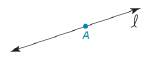Chapter 1.6, Problem 7E### Elementary Geometry for College St...

6th Edition
Daniel C. Alexander + 1 other
ISBN: 9781285195698

#### Solutions

Chapter
Section### Elementary Geometry for College St...

6th Edition
Daniel C. Alexander + 1 other
ISBN: 9781285195698
Textbook Problem
1 views

# In Exercise 5 to 9, use a compass and a straightedge to complete the constructions. Given: Line l containing point A Construct: A 45 ° angle with vertex ATo determine

To find:

The complete construction of A 45° angle with vertex at A.

Explanation

Given:

A point A is marked on the line l.

Construction:

The steps are given below for the complete construction.

a) Use the straightedge and extend line l from the point A to both the side of A and marked two point as B and B such that the length AB and AB should be equal.

b) Use B and B as the centers for the compass and take the radius greater than the length of BA.

c) These arcs intersects above the BB and the intersection point is marked as D

### Still sussing out bartleby?

Check out a sample textbook solution.

See a sample solution

#### The Solution to Your Study Problems

Bartleby provides explanations to thousands of textbook problems written by our experts, many with advanced degrees!

Get Started

#### A sample with a mean of M = 8 has X = 56. How many scores are in the sample?

Essentials of Statistics for The Behavioral Sciences (MindTap Course List)

#### In Exercises 1124, find the indicated limits, if they exist. 14. limx3x3x+4

Applied Calculus for the Managerial, Life, and Social Sciences: A Brief Approach

#### Calculate y'. 13. y=e1/xx2

Single Variable Calculus: Early Transcendentals, Volume I

#### It does not exist.

Study Guide for Stewart's Multivariable Calculus, 8th

#### For y = sin 6x, dydx=. a) cos 6x b) 6 cos x c) cos 6 d) 6 cos 6x

Study Guide for Stewart's Single Variable Calculus: Early Transcendentals, 8th